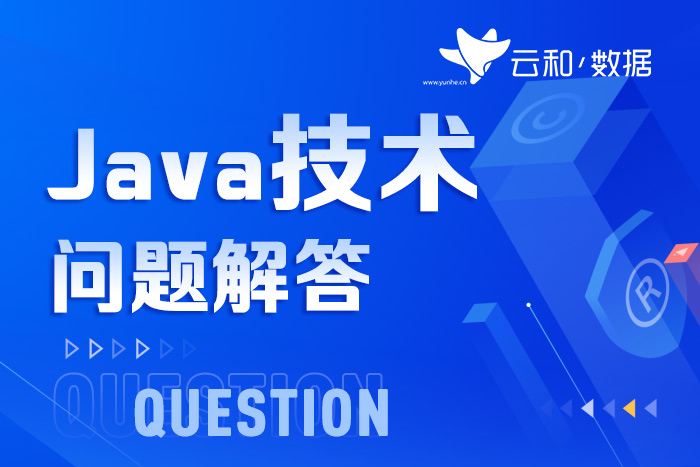• 华为
授权培训中心
• 腾讯云
一级认证培训中心
• 百度营销大学
豫陕深授权运营中心
• Oracle甲骨文
OAEP中心
• Microsoft Azure
微软云合作伙伴
• Unity公司
战略合作伙伴
• 普华基础软件
战略合作伙伴
• 新开普(股票代码300248)
旗下丹诚开普投资
• 中国互联网百强企业锐之旗
旗下锐旗资本投资

# JavaScript中形参和实参指的是什么？

• 发布时间：
2023-02-21
• 版权所有：
云和教育
• 分享：``````//功能1：求1~100的累加和
var sum = 0;
for (var i = 1; i <= 100; i++) {
sum te j;
}
console.log(sum);``````
``````//功能2：求10~50的累加和
var sun = 0;
for (var I = 10; i <= 50; i++) {
sum f = i;
}
console.log(sum);``````

``````//声明一个getSum函数，将代码写在大括号"{}”中
function getSum(numl, num2) {
var sum = 0;
for (var i = numl; i <= num2; i++) {
sum += i;
}
console.log(sum); //函数执行结束后，将结果输出
}
//调用getsum函数，在调用时需要写上小括号，并在小括号里传人参数
getSum(1， 100); // 输出结果:5050
getSum(10， 50); // 输出结果 :1230``````

• 集团官网
• 立即咨询
• QQ咨询
• 试听课程
• 咨询热线
请输入手机号立即免费通话
• 返回顶部